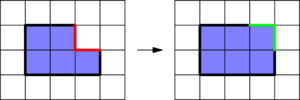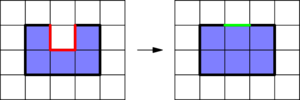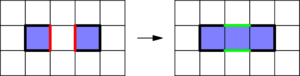# Internet Problem Solving Contest

## Solution to Problem A – Automated flooding

### Guessing the optimal solution for a square

A single isolated water cell does not spread at all.

With two water cells the best we can produce is obviously a 2 × 2 square: put the water cells diagonally adjacent to each other and wait for the water to spread.

After some experimentation it should be obvious that the best you can do with n water cells is an n × n square. Or, as seen from the other side, an optimal solution for an n × n square seems to involve n manually placed water cells.

In particular, one optimal solution for a square is to change one of its diagonals into water. The water will then spread to adjacent diagonals, eventually covering the entire square:

``````W....        WW...        WWW..        WWWW.        WWWWW
.W...        WWW..        WWWW.        WWWWW        WWWWW
..W..   ->   .WWW.   ->   WWWWW   ->   WWWWW   ->   WWWWW
...W.        ..WWW        .WWWW        WWWWW        WWWWW
....W        ...WW        ..WWW        .WWWW        WWWWW
``````

Of course, this is by far not the only optimal pattern that works. For example, a 4 × 4 square can also be optimally flooded like this:

``````.W..
W...
...W
..W.``````

Still, the task was to find any one optimal pattern, and the diagonal is almost certainly the easiest one to implement.

### Proof of optimality

The cutest part of this problem is that there is a really pretty proof that the solution shown above is optimal. This proof does also work for rectangles.

The key observation is to look at the circumference of the flooded area. Remember that a cell gets auto-flooded if and only if at least two of its neighbors are already water cells. This means that whenever a cell gets flooded, the circumference of the flooded area cannot increase. If the newly flooded cell had two neighbors, the circumference stays the same, if it had more neighbors, the circumference decreases.

At the end of the automated flooding process the entire rectangle should be covered by water. The circumference of the rectangle is 2(r + c). Therefore, any valid set of manually selected cells must have a circumference of at least 2(r + c). And as each click increases the circumference of the flooded area by at most 4, we need at least 2(r + c)/4 clicks.

If the rectangle is an n × n square, the above proof tells us that we need at least n clicks. Hence, any solution that uses exactly n clicks, including the one presented above, is indeed optimal.

Below are a few examples of how the circumference changes when the water spreads.### Dealing with rectangles

From the proof shown above we know that in the general case we need at least ⌈(r + c)/2⌉ clicks. All that remains is to show that patterns with this exact number of clicks always exist.

One possible construction looks as follows: Without loss of generality, let’s assume that r < c and that c = r + d. The minimum number of clicks can now be rewritten as r + ⌈d/2⌉. Thus, we can use r clicks to fill an r × r square, and another d/2⌉ clicks to fill the remaining d columns.

Here is one such pattern: even d on the left, odd d on the right.

``````W........      W.........
.W.......      .W........
..W......      ..W.......
...W.....      ...W......
....W.W.W      ....W.W.WW``````

We can easily verify that this pattern fills the entire rectangle with water, and from the proof we know that the number of clicks is optimal, q.e.d.